Скачать презентацию Handout 1 SI 760 EECS 597

• Количество слайдов: 57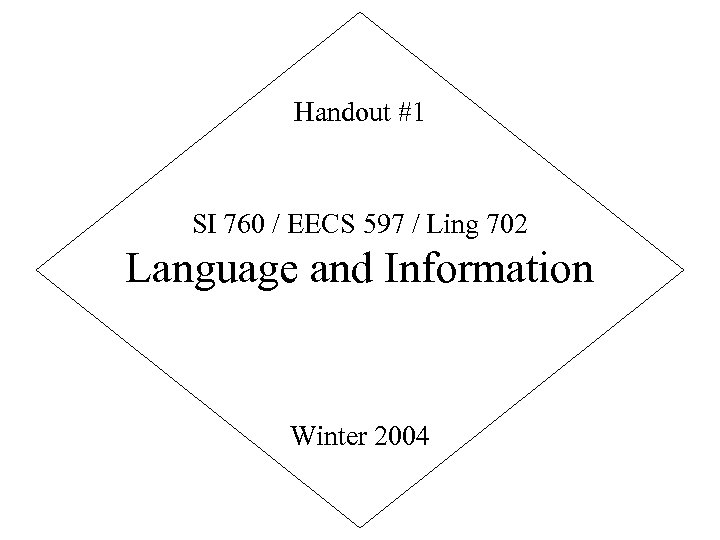Handout #1 SI 760 / EECS 597 / Ling 702 Language and Information Winter 2004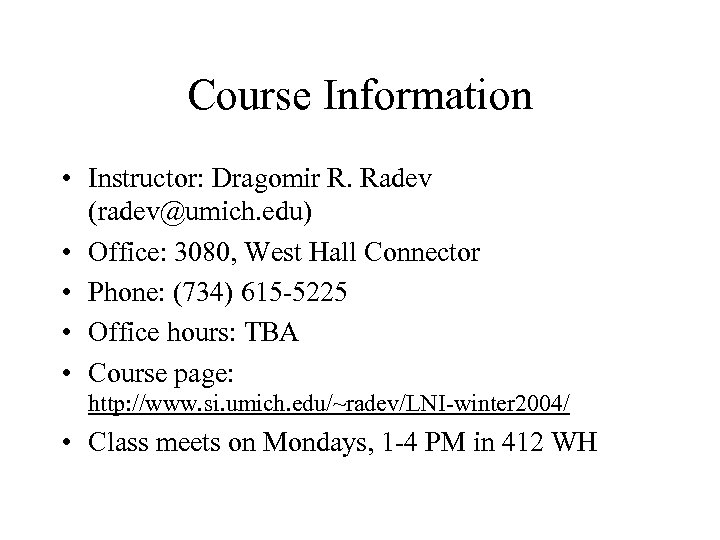Course Information • Instructor: Dragomir R. Radev ([email protected] edu) • Office: 3080, West Hall Connector • Phone: (734) 615 -5225 • Office hours: TBA • Course page: http: //www. si. umich. edu/~radev/LNI-winter 2004/ • Class meets on Mondays, 1 -4 PM in 412 WH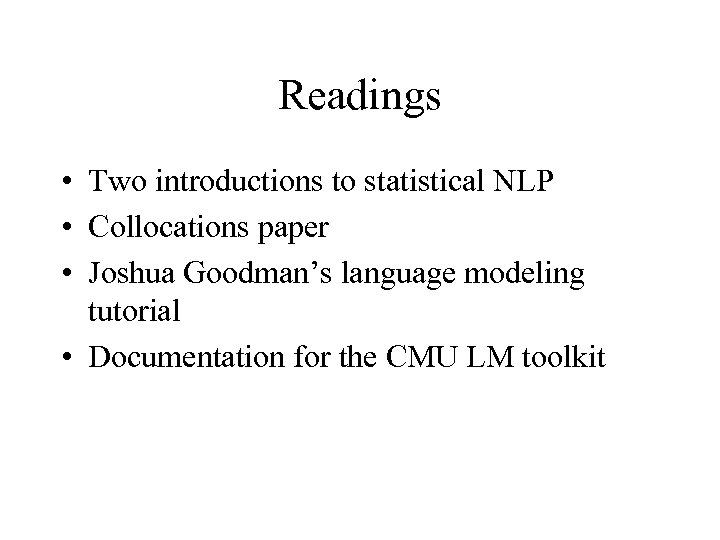Readings • Two introductions to statistical NLP • Collocations paper • Joshua Goodman’s language modeling tutorial • Documentation for the CMU LM toolkit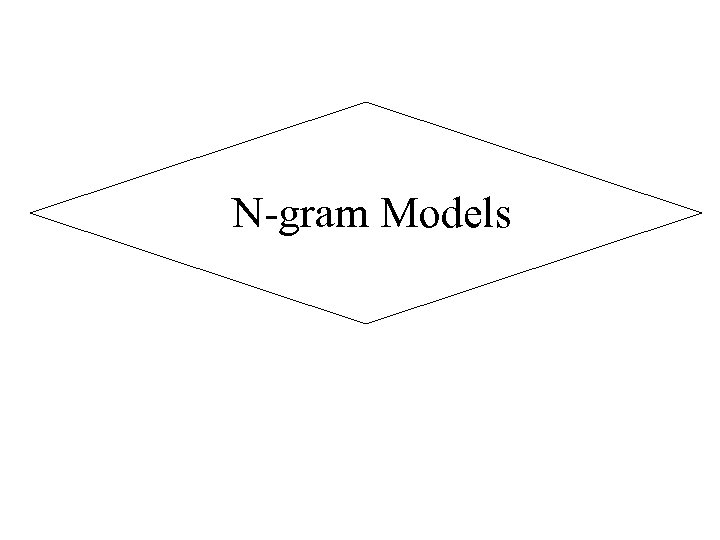N-gram Models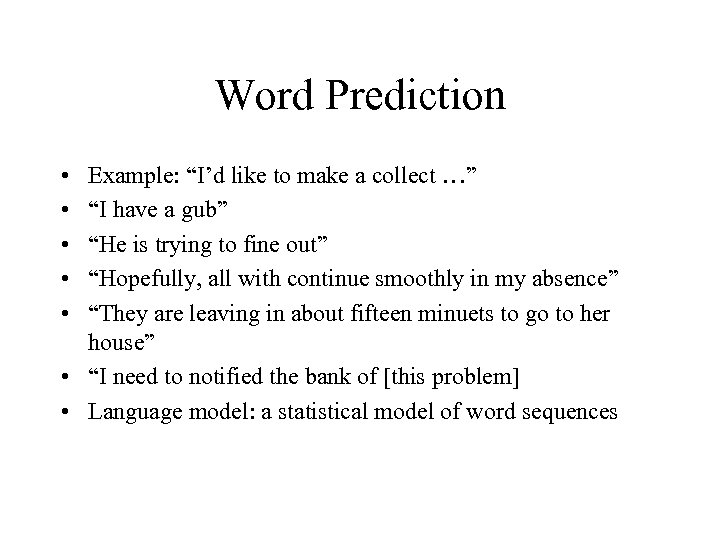Word Prediction • • • Example: “I’d like to make a collect …” “I have a gub” “He is trying to fine out” “Hopefully, all with continue smoothly in my absence” “They are leaving in about fifteen minuets to go to her house” • “I need to notified the bank of [this problem] • Language model: a statistical model of word sequences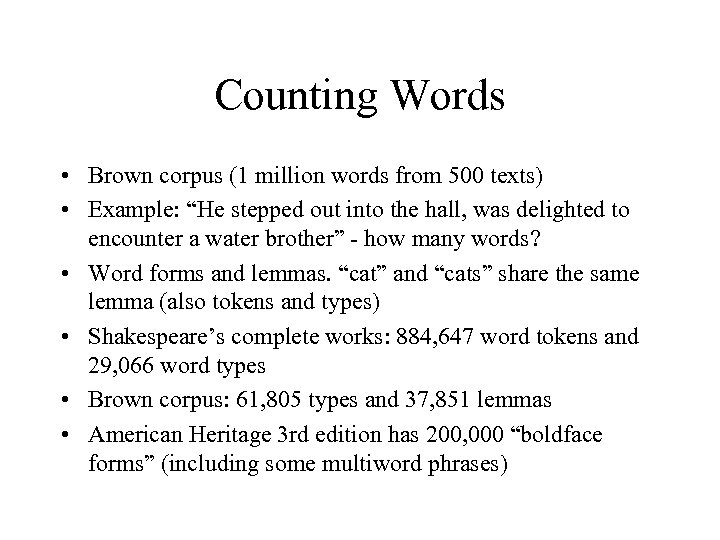Counting Words • Brown corpus (1 million words from 500 texts) • Example: “He stepped out into the hall, was delighted to encounter a water brother” - how many words? • Word forms and lemmas. “cat” and “cats” share the same lemma (also tokens and types) • Shakespeare’s complete works: 884, 647 word tokens and 29, 066 word types • Brown corpus: 61, 805 types and 37, 851 lemmas • American Heritage 3 rd edition has 200, 000 “boldface forms” (including some multiword phrases)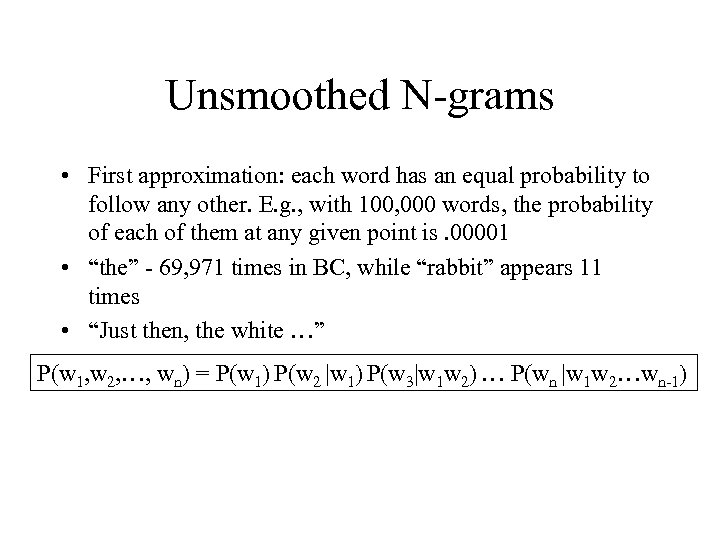Unsmoothed N-grams • First approximation: each word has an equal probability to follow any other. E. g. , with 100, 000 words, the probability of each of them at any given point is. 00001 • “the” - 69, 971 times in BC, while “rabbit” appears 11 times • “Just then, the white …” P(w 1, w 2, …, wn) = P(w 1) P(w 2 |w 1) P(w 3|w 1 w 2) … P(wn |w 1 w 2…wn-1)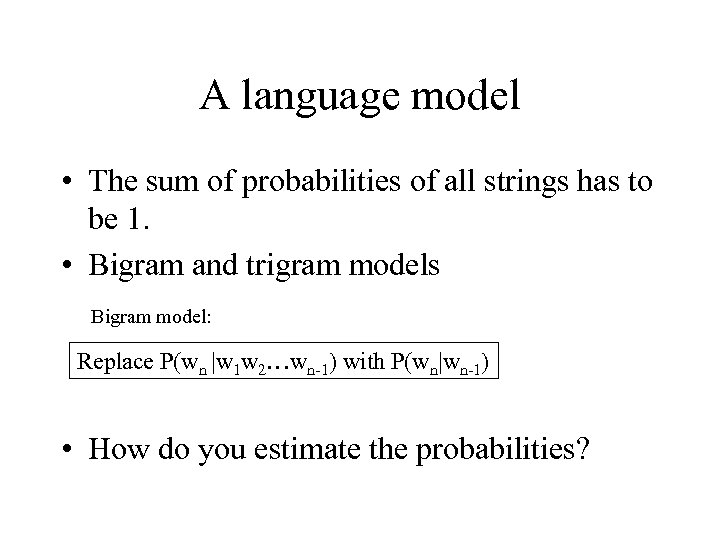A language model • The sum of probabilities of all strings has to be 1. • Bigram and trigram models Bigram model: Replace P(wn |w 1 w 2…wn-1) with P(wn|wn-1) • How do you estimate the probabilities?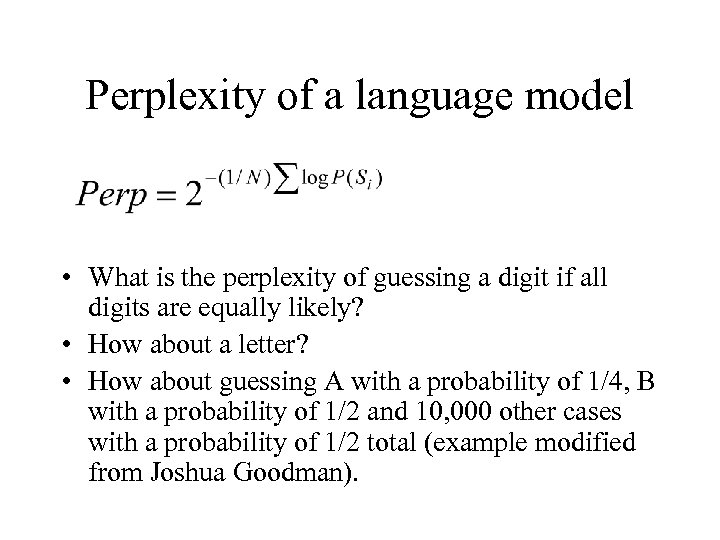Perplexity of a language model • What is the perplexity of guessing a digit if all digits are equally likely? • How about a letter? • How about guessing A with a probability of 1/4, B with a probability of 1/2 and 10, 000 other cases with a probability of 1/2 total (example modified from Joshua Goodman).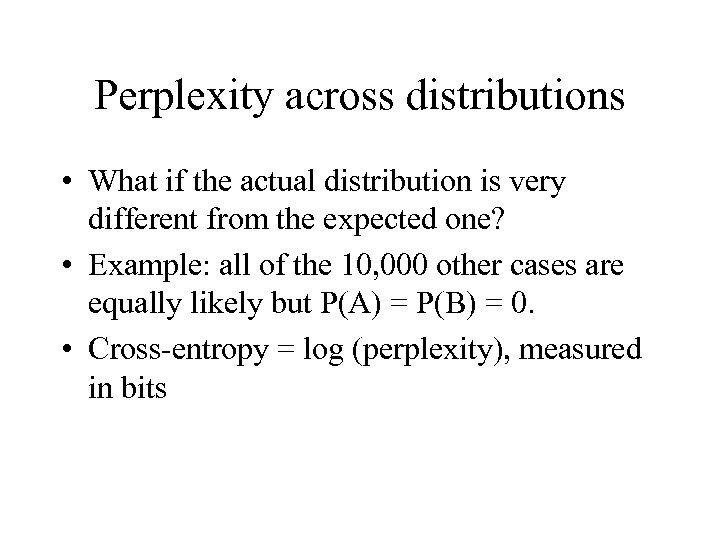Perplexity across distributions • What if the actual distribution is very different from the expected one? • Example: all of the 10, 000 other cases are equally likely but P(A) = P(B) = 0. • Cross-entropy = log (perplexity), measured in bits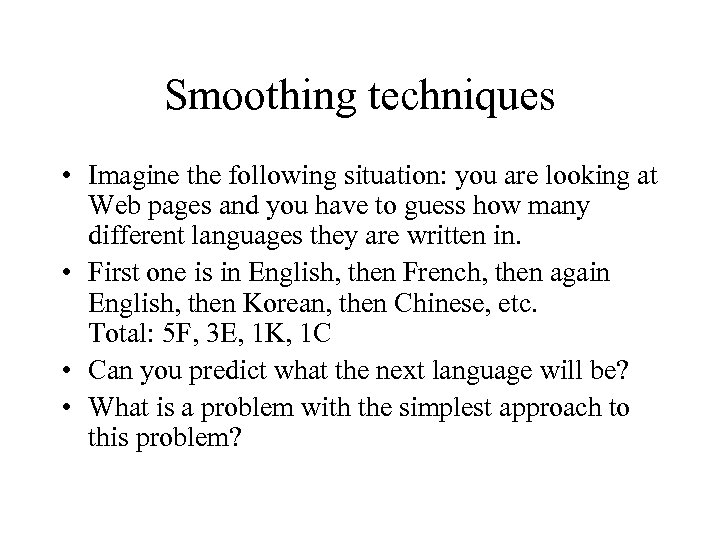Smoothing techniques • Imagine the following situation: you are looking at Web pages and you have to guess how many different languages they are written in. • First one is in English, then French, then again English, then Korean, then Chinese, etc. Total: 5 F, 3 E, 1 K, 1 C • Can you predict what the next language will be? • What is a problem with the simplest approach to this problem?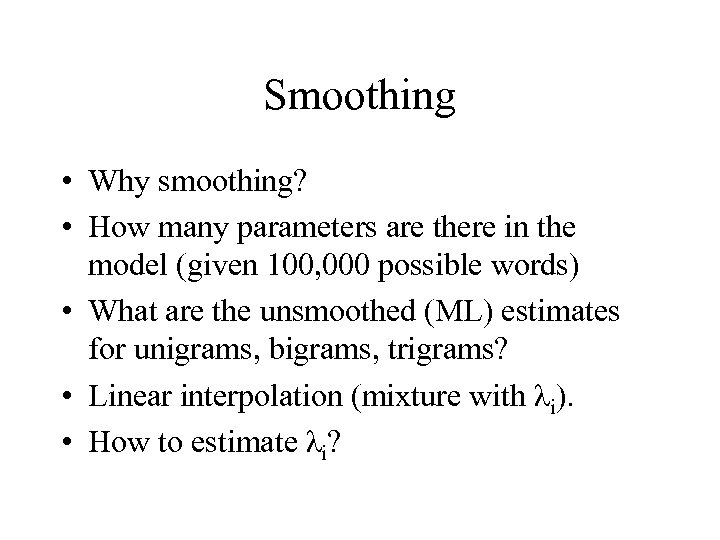Smoothing • Why smoothing? • How many parameters are there in the model (given 100, 000 possible words) • What are the unsmoothed (ML) estimates for unigrams, bigrams, trigrams? • Linear interpolation (mixture with λi). • How to estimate λi?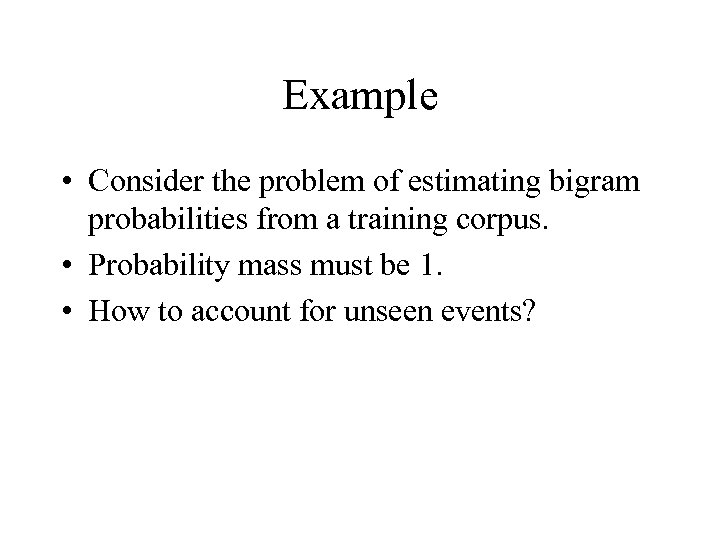Example • Consider the problem of estimating bigram probabilities from a training corpus. • Probability mass must be 1. • How to account for unseen events?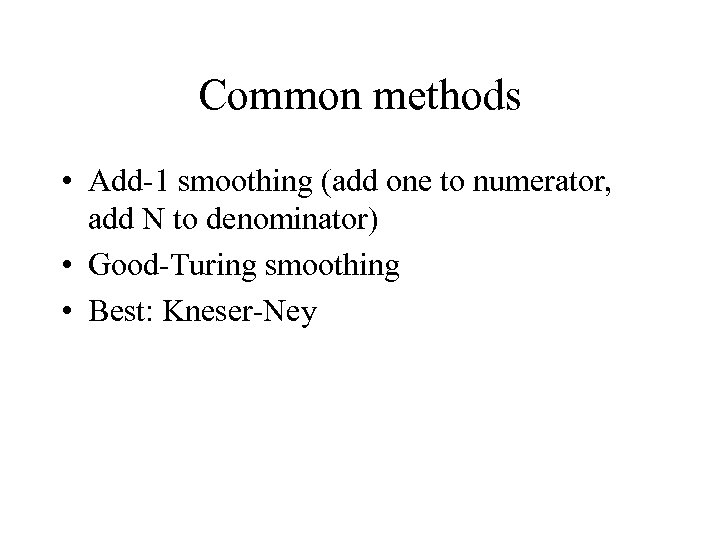Common methods • Add-1 smoothing (add one to numerator, add N to denominator) • Good-Turing smoothing • Best: Kneser-Ney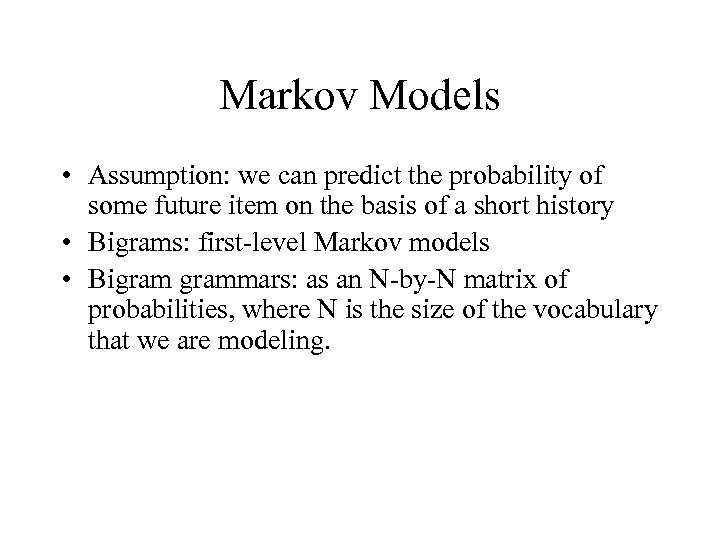Markov Models • Assumption: we can predict the probability of some future item on the basis of a short history • Bigrams: first-level Markov models • Bigrammars: as an N-by-N matrix of probabilities, where N is the size of the vocabulary that we are modeling.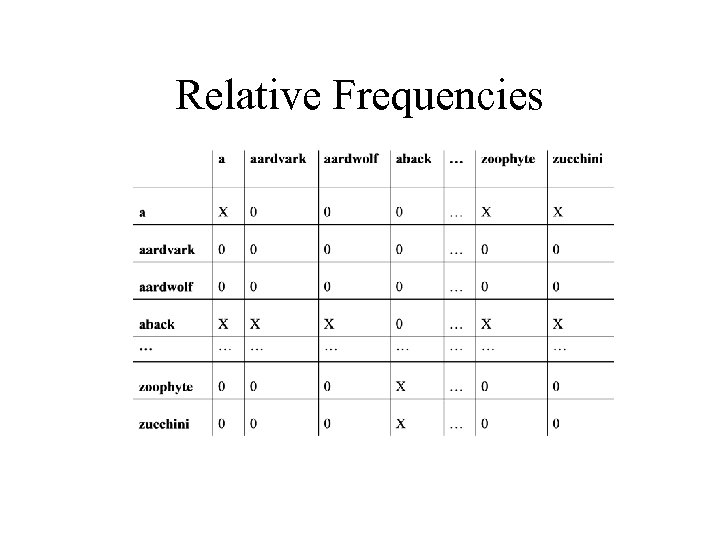Relative Frequencies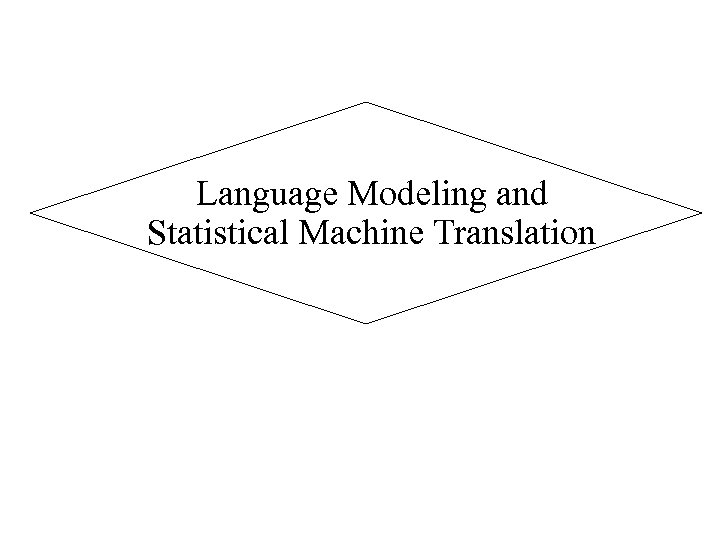Language Modeling and Statistical Machine Translation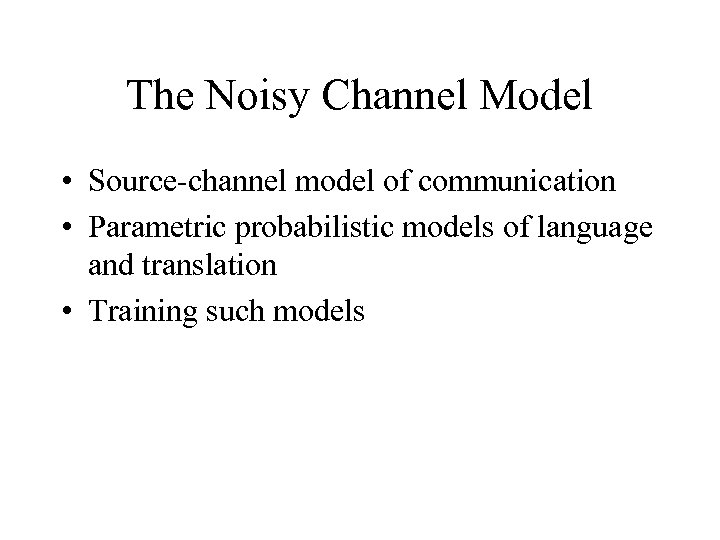The Noisy Channel Model • Source-channel model of communication • Parametric probabilistic models of language and translation • Training such models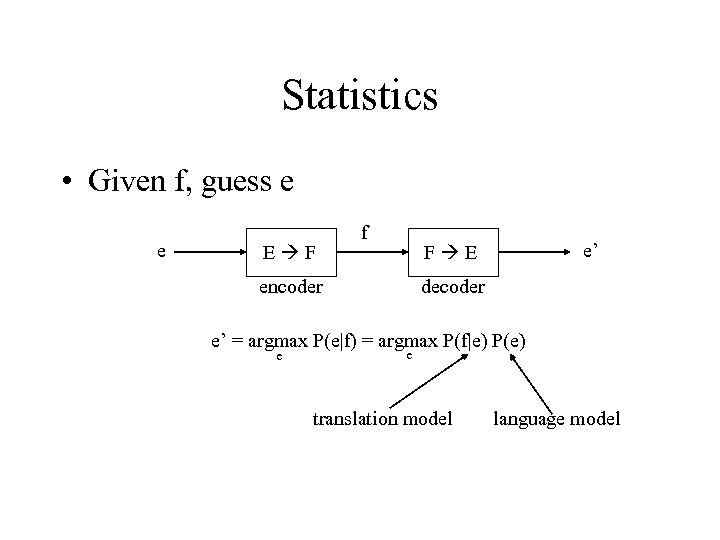Statistics • Given f, guess e e E F f e’ F E encoder decoder e’ = argmax P(e|f) = argmax P(f|e) P(e) e e translation model language model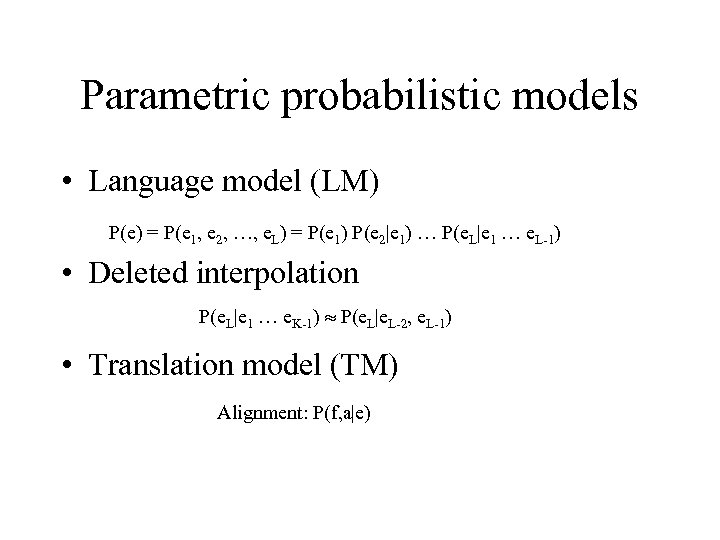Parametric probabilistic models • Language model (LM) P(e) = P(e 1, e 2, …, e. L) = P(e 1) P(e 2|e 1) … P(e. L|e 1 … e. L-1) • Deleted interpolation P(e. L|e 1 … e. K-1) P(e. L|e. L-2, e. L-1) • Translation model (TM) Alignment: P(f, a|e)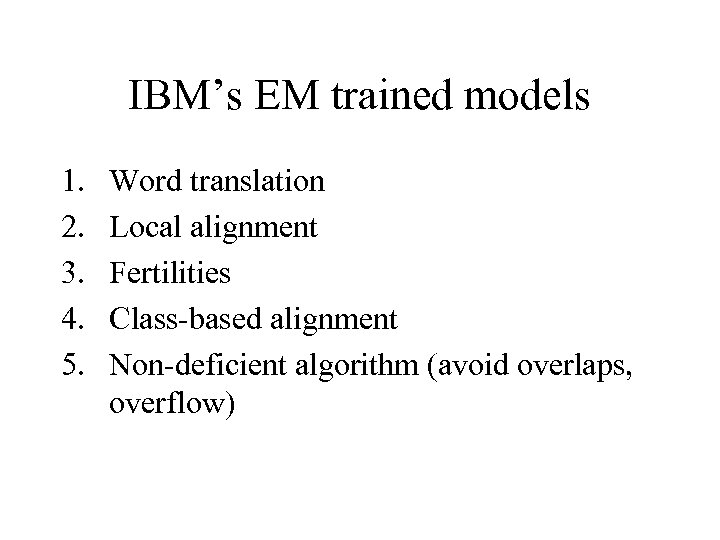IBM’s EM trained models 1. 2. 3. 4. 5. Word translation Local alignment Fertilities Class-based alignment Non-deficient algorithm (avoid overlaps, overflow)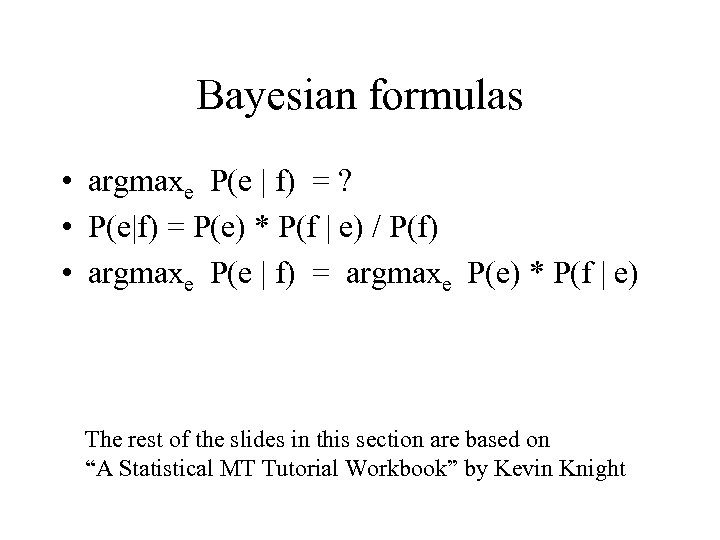Bayesian formulas • argmaxe P(e | f) = ? • P(e|f) = P(e) * P(f | e) / P(f) • argmaxe P(e | f) = argmaxe P(e) * P(f | e) The rest of the slides in this section are based on “A Statistical MT Tutorial Workbook” by Kevin Knight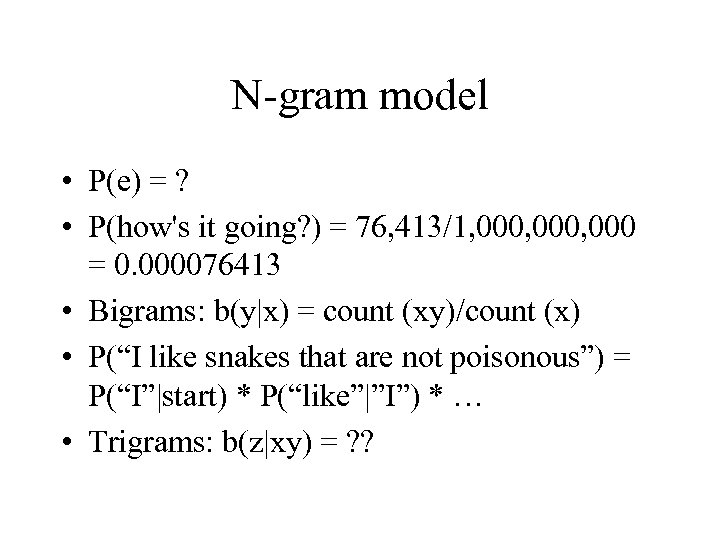N-gram model • P(e) = ? • P(how's it going? ) = 76, 413/1, 000, 000 = 0. 000076413 • Bigrams: b(y|x) = count (xy)/count (x) • P(“I like snakes that are not poisonous”) = P(“I”|start) * P(“like”|”I”) * … • Trigrams: b(z|xy) = ? ?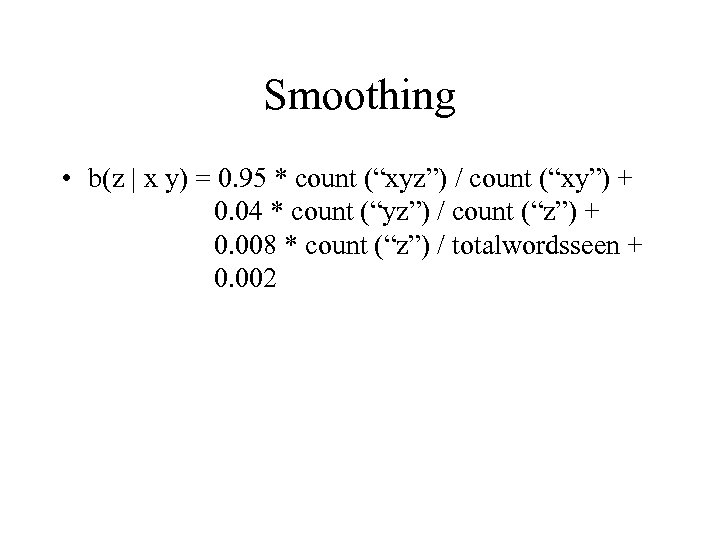Smoothing • b(z | x y) = 0. 95 * count (“xyz”) / count (“xy”) + 0. 04 * count (“yz”) / count (“z”) + 0. 008 * count (“z”) / totalwordsseen + 0. 002Ordering words (1) a a centre earthquake evacuation forced has historic Italian of of second southern strong the village (2) met Paul Wendy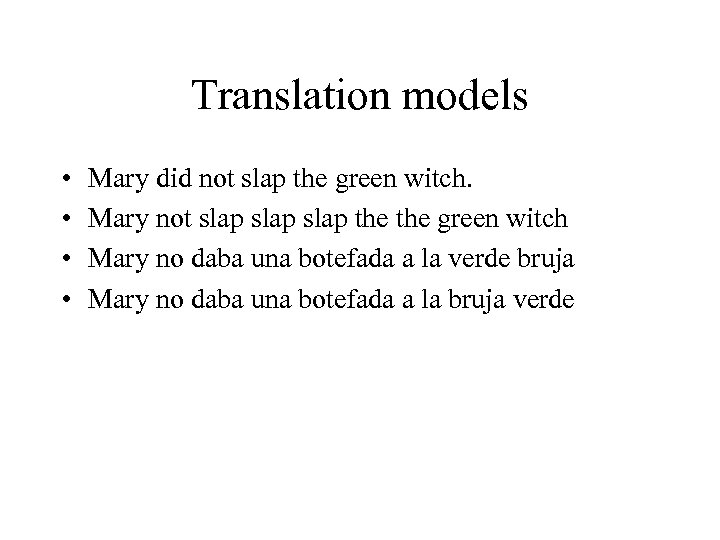Translation models • • Mary did not slap the green witch. Mary not slap the green witch Mary no daba una botefada a la verde bruja Mary no daba una botefada a la bruja verde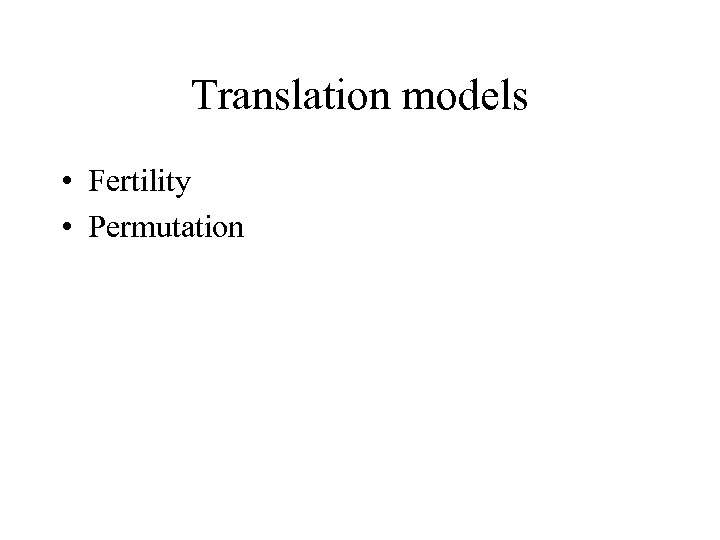Translation models • Fertility • Permutation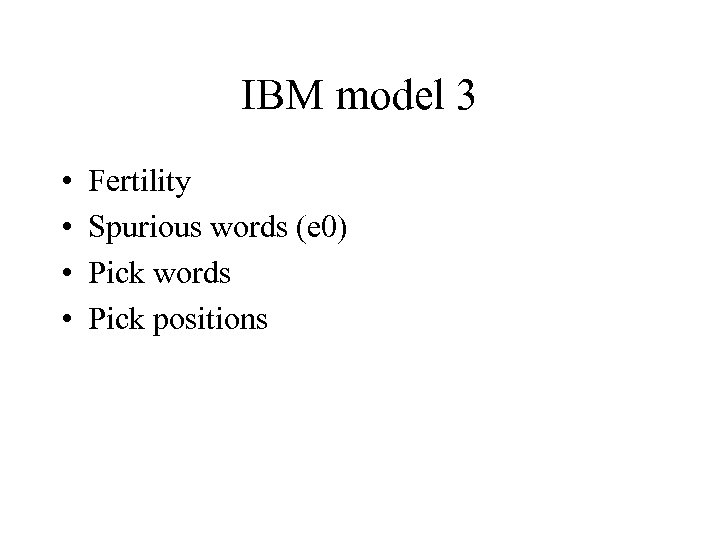IBM model 3 • • Fertility Spurious words (e 0) Pick words Pick positions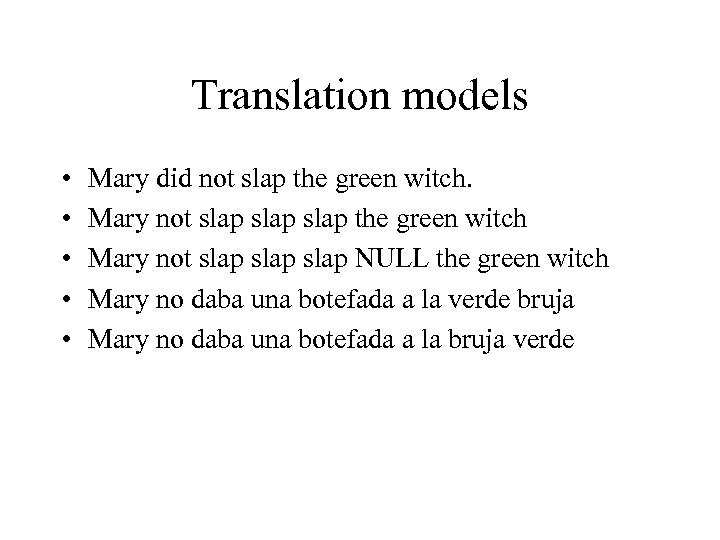Translation models • • • Mary did not slap the green witch. Mary not slap the green witch Mary not slap NULL the green witch Mary no daba una botefada a la verde bruja Mary no daba una botefada a la bruja verde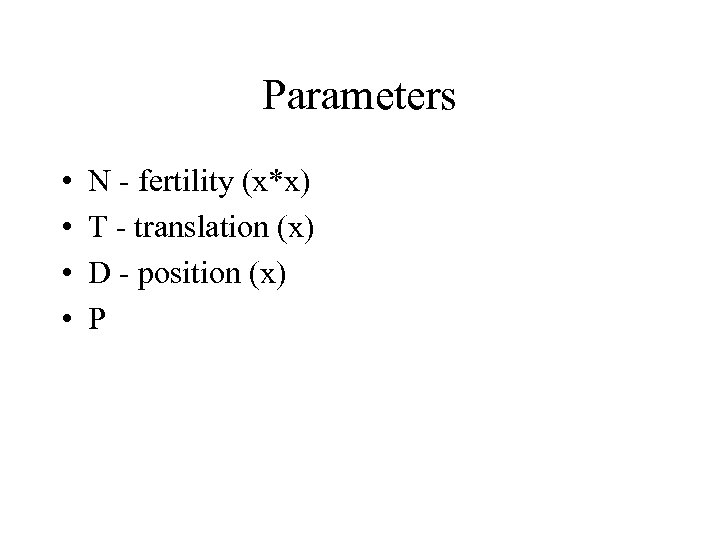Parameters • • N - fertility (x*x) T - translation (x) D - position (x) P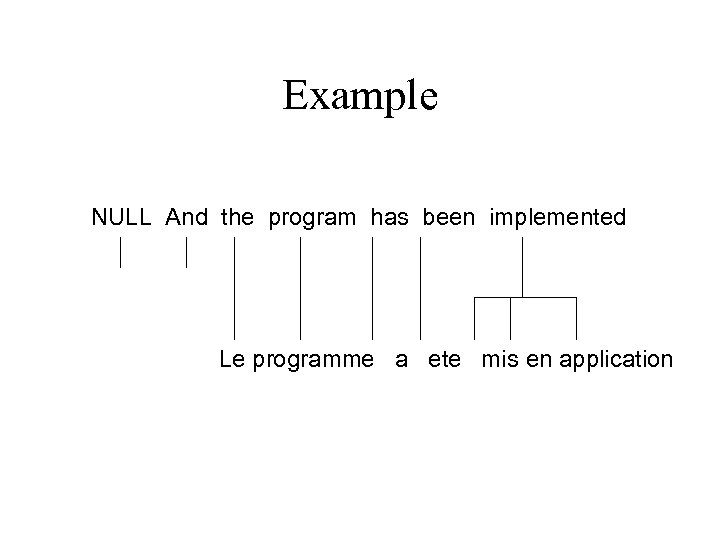Example NULL And the program has been implemented Le programme a ete mis en application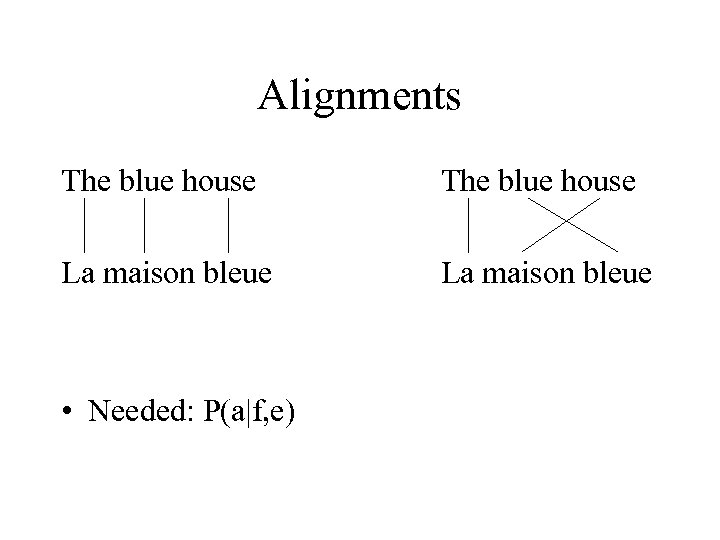Alignments The blue house La maison bleue • Needed: P(a|f, e)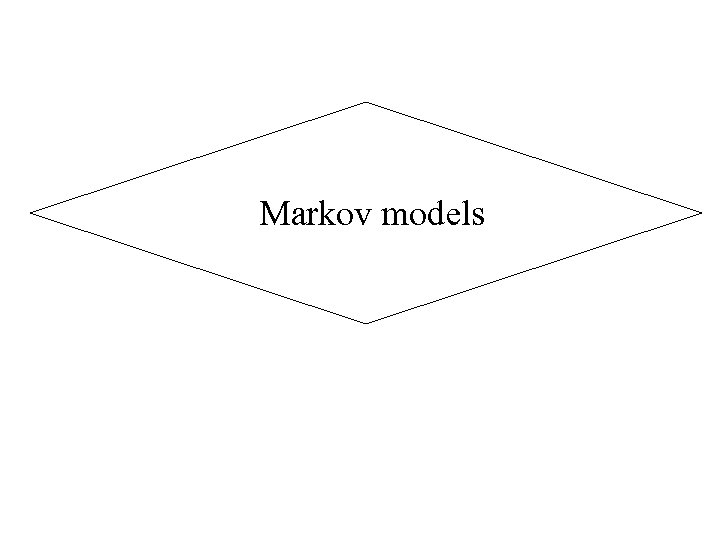Markov models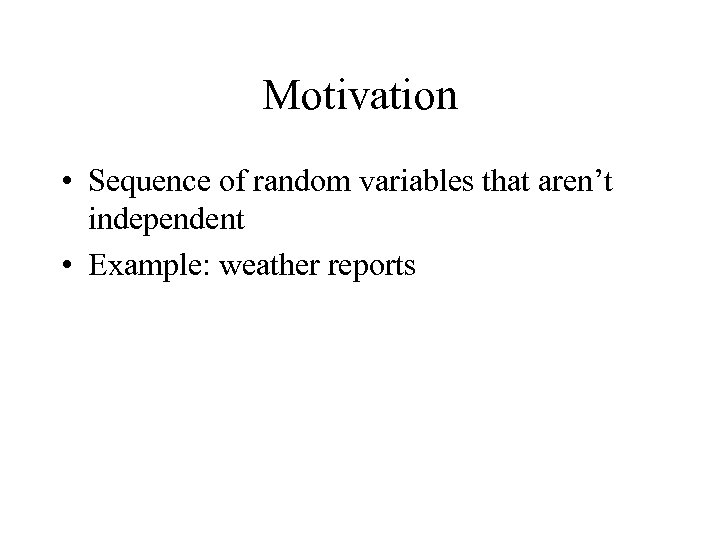Motivation • Sequence of random variables that aren’t independent • Example: weather reports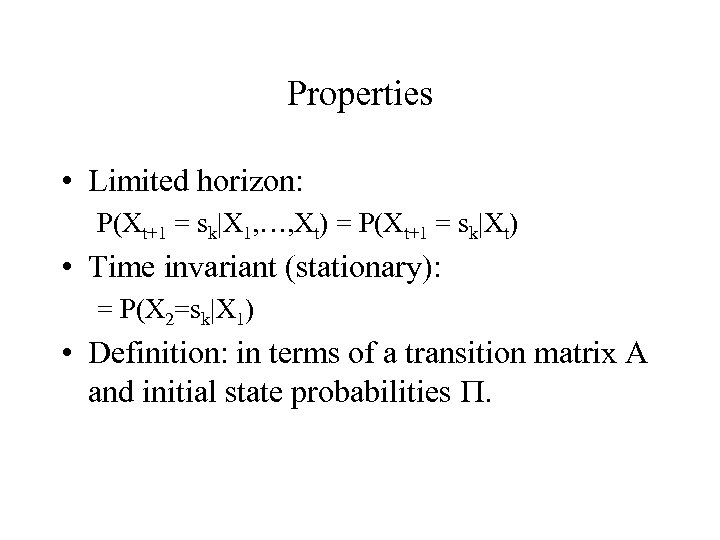Properties • Limited horizon: P(Xt+1 = sk|X 1, …, Xt) = P(Xt+1 = sk|Xt) • Time invariant (stationary): = P(X 2=sk|X 1) • Definition: in terms of a transition matrix A and initial state probabilities .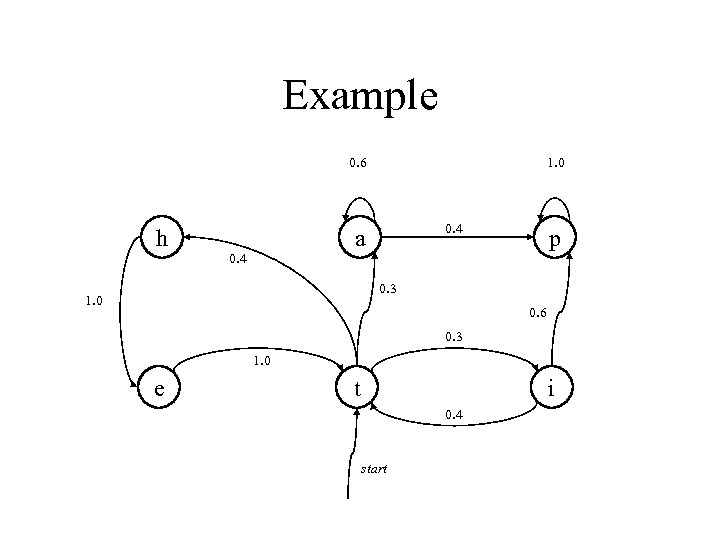Example 0. 6 h 1. 0 0. 4 a 0. 4 p 0. 3 1. 0 0. 6 0. 3 1. 0 e t i 0. 4 start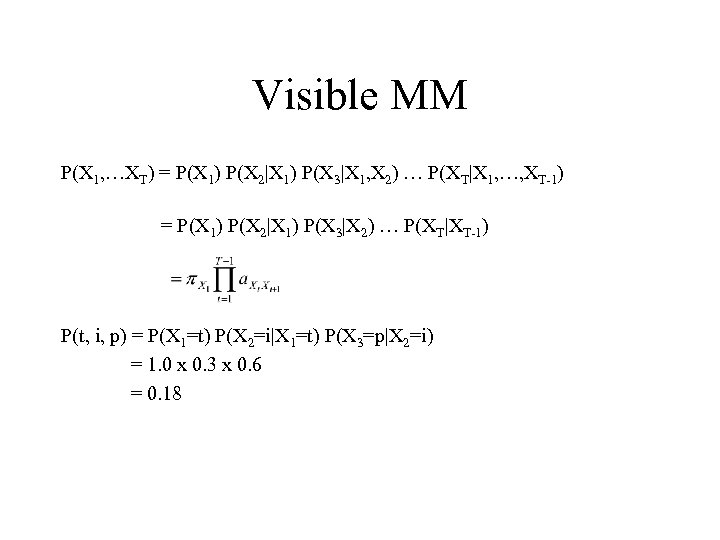Visible MM P(X 1, …XT) = P(X 1) P(X 2|X 1) P(X 3|X 1, X 2) … P(XT|X 1, …, XT-1) = P(X 1) P(X 2|X 1) P(X 3|X 2) … P(XT|XT-1) P(t, i, p) = P(X 1=t) P(X 2=i|X 1=t) P(X 3=p|X 2=i) = 1. 0 x 0. 3 x 0. 6 = 0. 18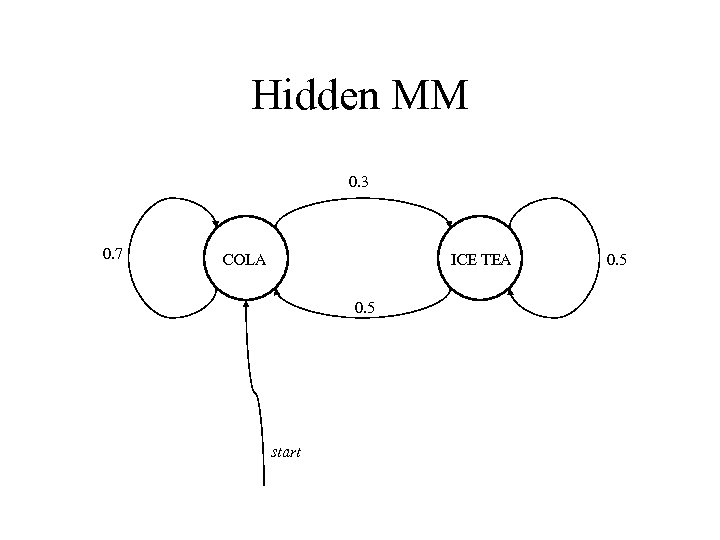Hidden MM 0. 3 0. 7 COLA ICE TEA 0. 5 start 0. 5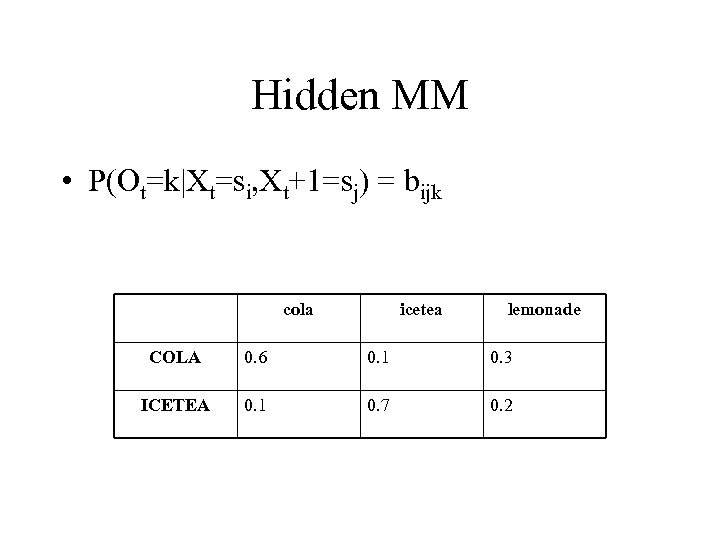Hidden MM • P(Ot=k|Xt=si, Xt+1=sj) = bijk cola icetea lemonade COLA 0. 6 0. 1 0. 3 ICETEA 0. 1 0. 7 0. 2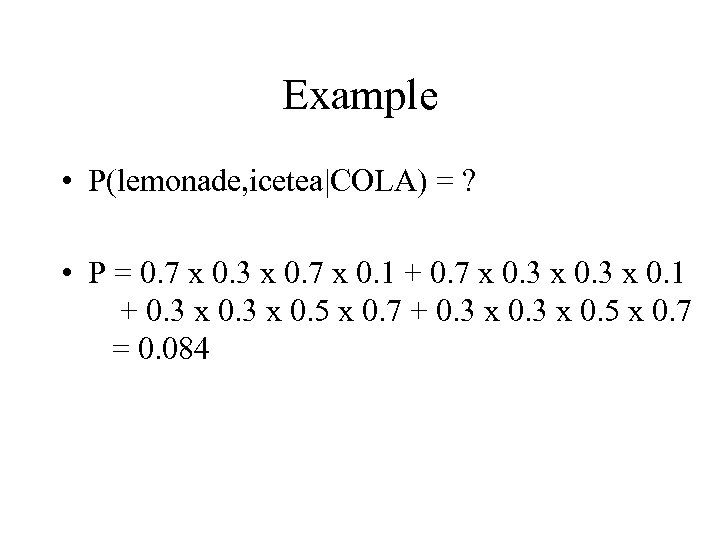Example • P(lemonade, icetea|COLA) = ? • P = 0. 7 x 0. 3 x 0. 7 x 0. 1 + 0. 7 x 0. 3 x 0. 1 + 0. 3 x 0. 5 x 0. 7 = 0. 084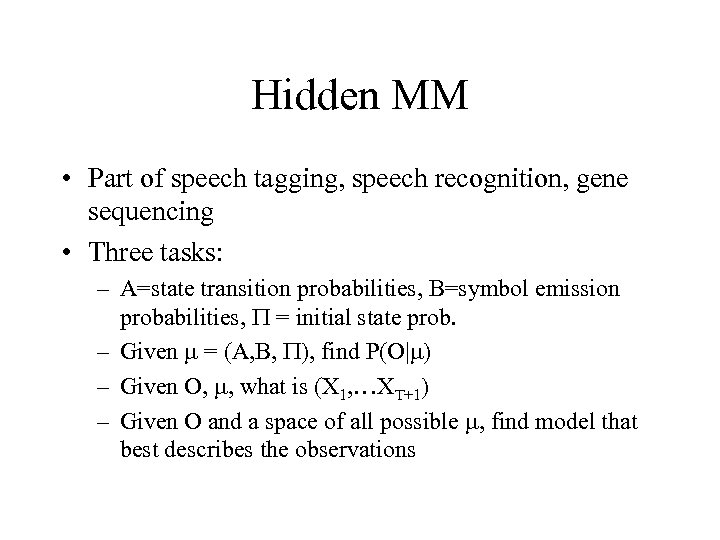Hidden MM • Part of speech tagging, speech recognition, gene sequencing • Three tasks: – A=state transition probabilities, B=symbol emission probabilities, = initial state prob. – Given = (A, B, ), find P(O| ) – Given O, , what is (X 1, …XT+1) – Given O and a space of all possible , find model that best describes the observations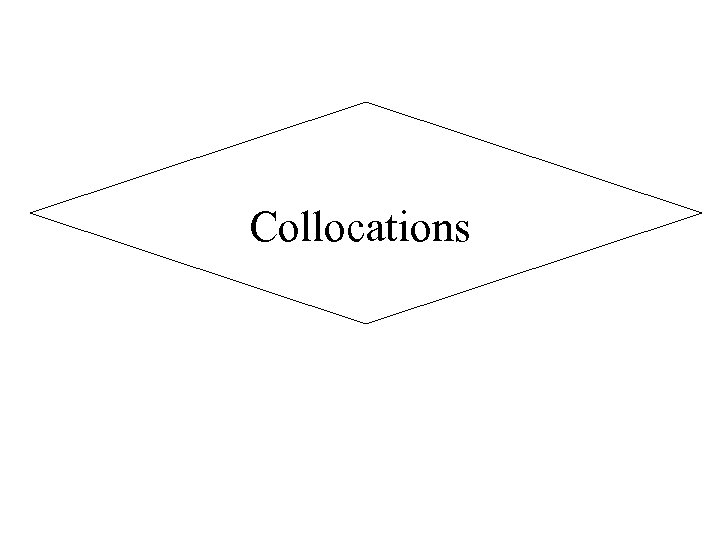Collocations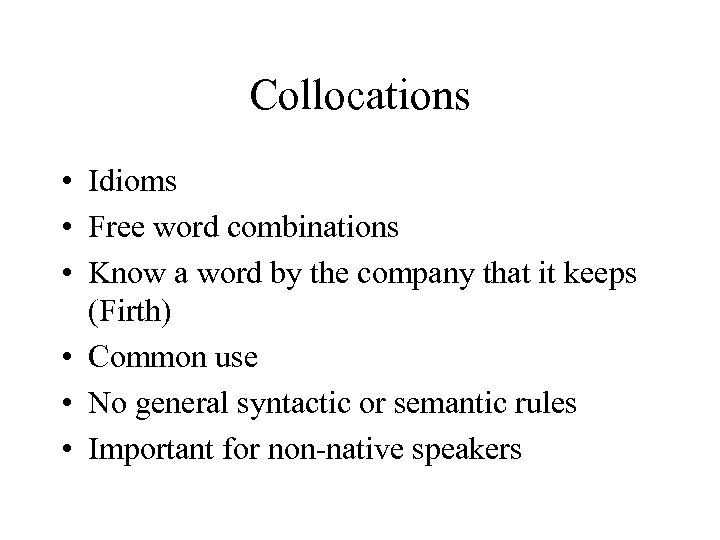Collocations • Idioms • Free word combinations • Know a word by the company that it keeps (Firth) • Common use • No general syntactic or semantic rules • Important for non-native speakers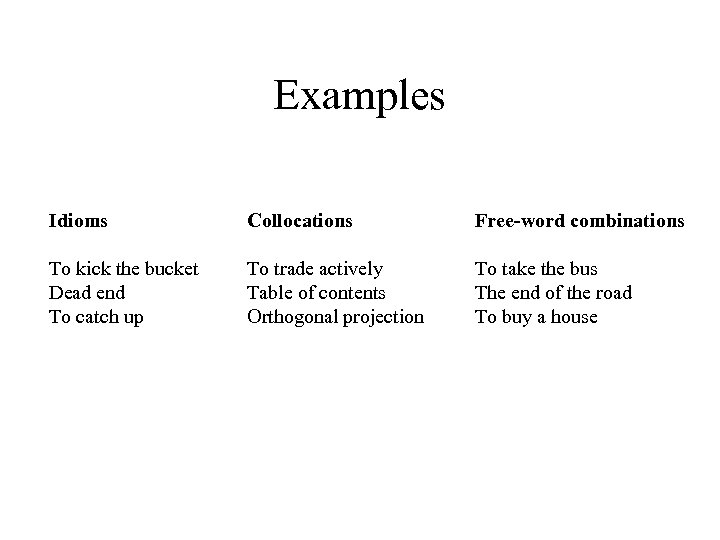Examples Idioms Collocations Free-word combinations To kick the bucket Dead end To catch up To trade actively Table of contents Orthogonal projection To take the bus The end of the road To buy a house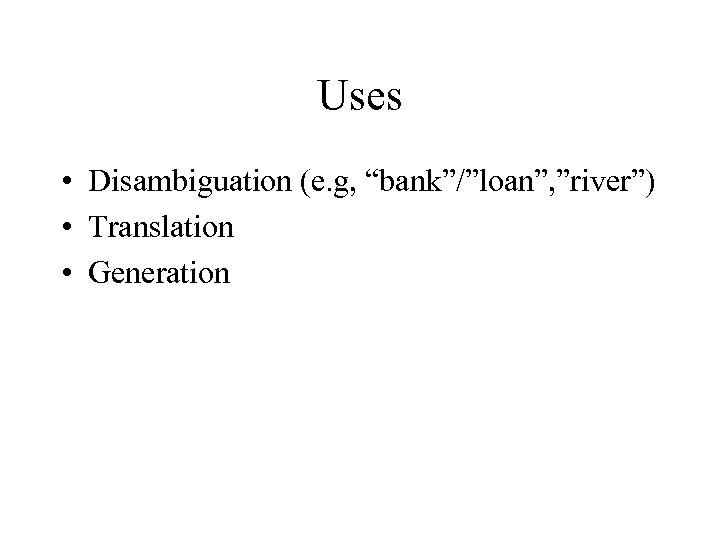Uses • Disambiguation (e. g, “bank”/”loan”, ”river”) • Translation • Generation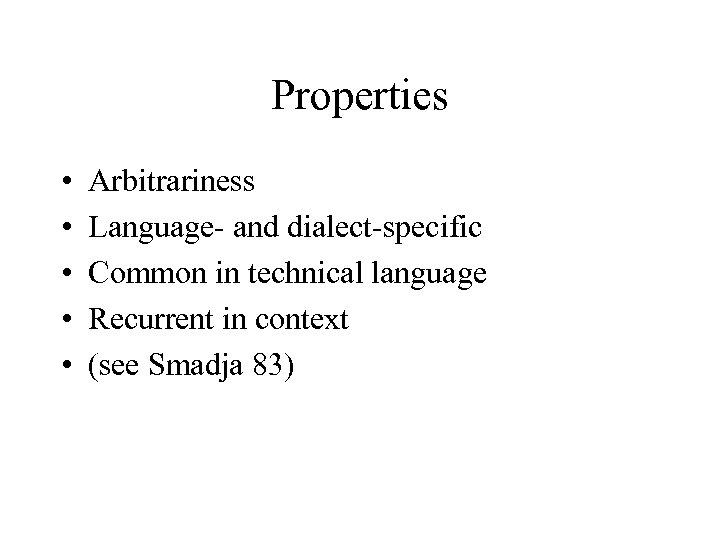Properties • • • Arbitrariness Language- and dialect-specific Common in technical language Recurrent in context (see Smadja 83)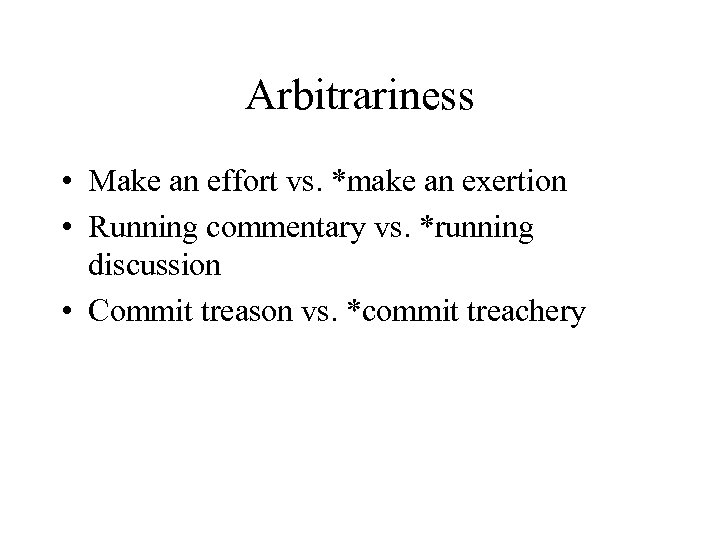Arbitrariness • Make an effort vs. *make an exertion • Running commentary vs. *running discussion • Commit treason vs. *commit treachery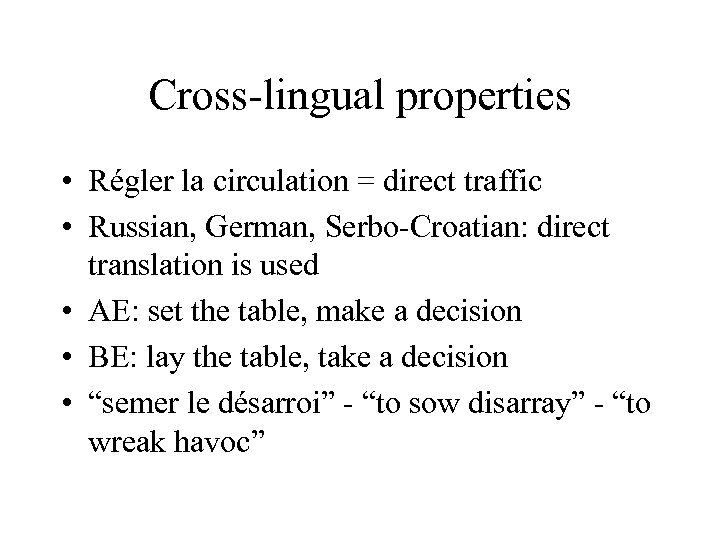Cross-lingual properties • Régler la circulation = direct traffic • Russian, German, Serbo-Croatian: direct translation is used • AE: set the table, make a decision • BE: lay the table, take a decision • “semer le désarroi” - “to sow disarray” - “to wreak havoc”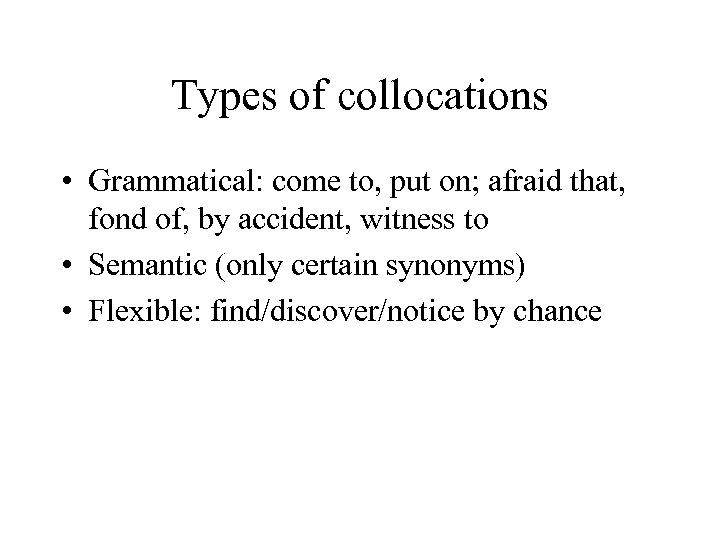Types of collocations • Grammatical: come to, put on; afraid that, fond of, by accident, witness to • Semantic (only certain synonyms) • Flexible: find/discover/notice by chance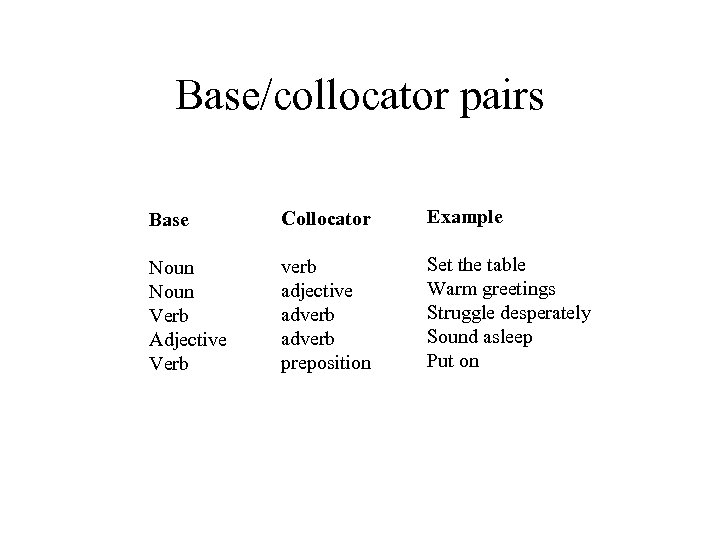Base/collocator pairs Base Collocator Example Noun Verb Adjective Verb verb adjective adverb preposition Set the table Warm greetings Struggle desperately Sound asleep Put on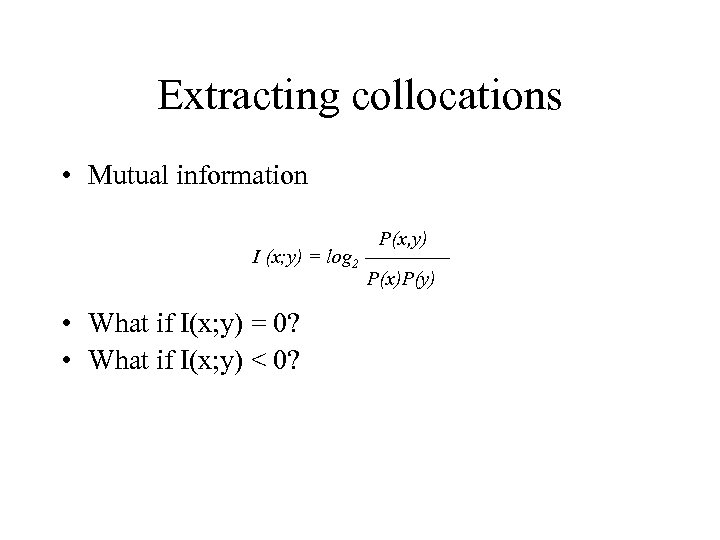Extracting collocations • Mutual information I (x; y) = log 2 • What if I(x; y) = 0? • What if I(x; y) < 0? P(x, y) P(x)P(y)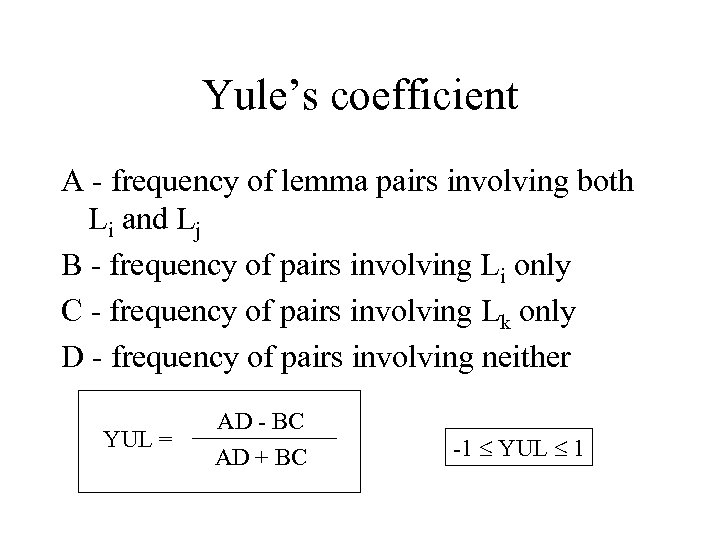Yule’s coefficient A - frequency of lemma pairs involving both Li and Lj B - frequency of pairs involving Li only C - frequency of pairs involving Lk only D - frequency of pairs involving neither YUL = AD - BC AD + BC -1 YUL 1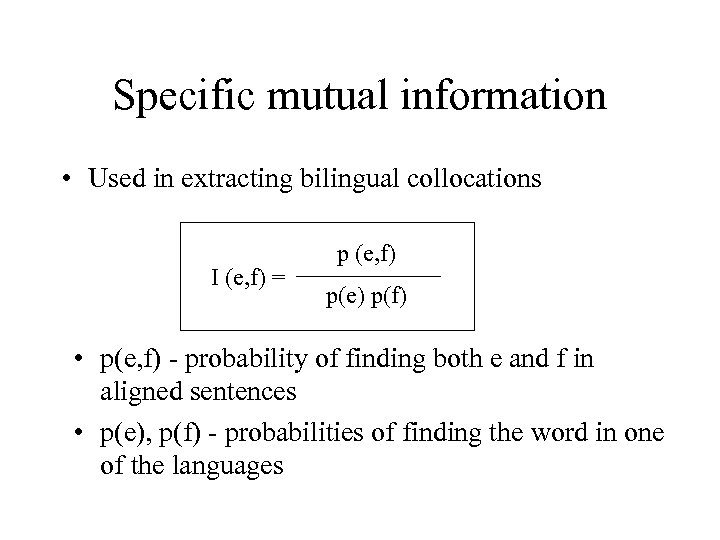Specific mutual information • Used in extracting bilingual collocations I (e, f) = p (e, f) p(e) p(f) • p(e, f) - probability of finding both e and f in aligned sentences • p(e), p(f) - probabilities of finding the word in one of the languages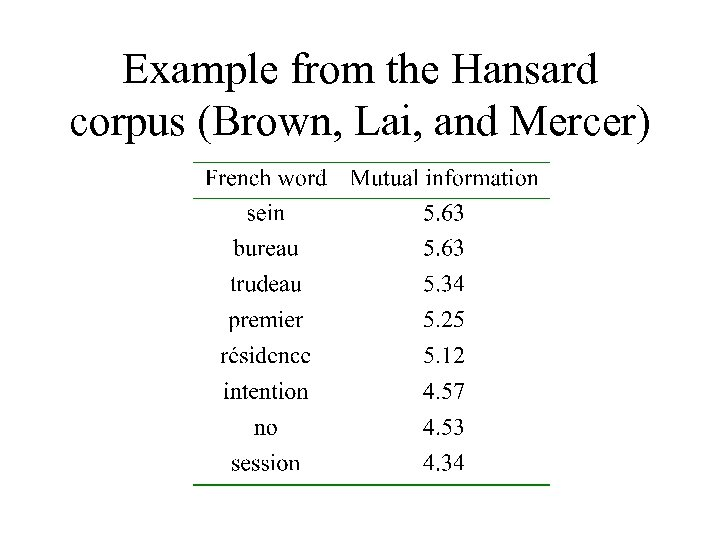Example from the Hansard corpus (Brown, Lai, and Mercer)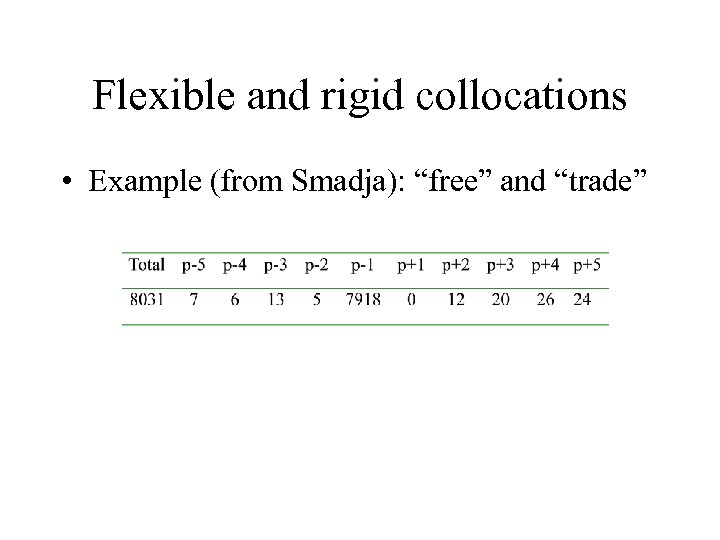Flexible and rigid collocations • Example (from Smadja): “free” and “trade”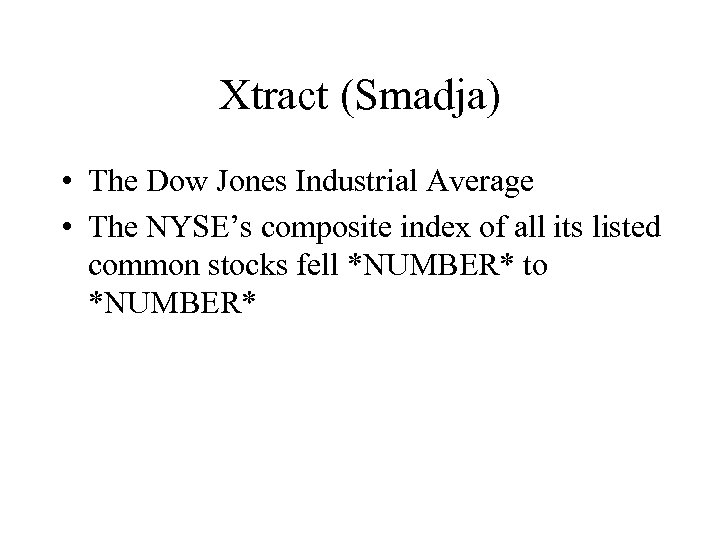Xtract (Smadja) • The Dow Jones Industrial Average • The NYSE’s composite index of all its listed common stocks fell *NUMBER* to *NUMBER*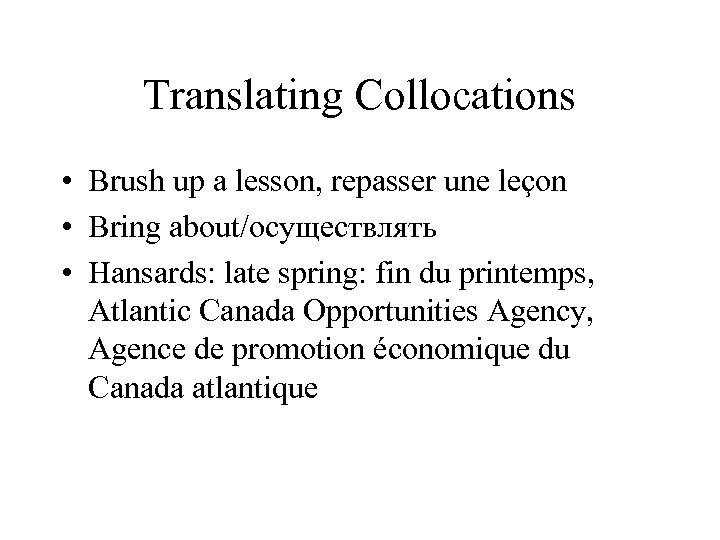Translating Collocations • Brush up a lesson, repasser une leçon • Bring about/осуществлять • Hansards: late spring: fin du printemps, Atlantic Canada Opportunities Agency, Agence de promotion économique du Canada atlantique